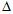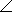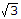# Electrical Engineering - Three-Phase Systems in Power Applications

### Exercise :: Three-Phase Systems in Power Applications - General Questions

16.

In a certain Y-Y system, the source phase currents each have a magnitude of 9 A. The magnitude of each load current for a balanced load condition is

 A. 3 A B. 6 A C. 9 A D. 27 A

Explanation:

No answer description available for this question. Let us discuss.

17.

A three-phase-connected generator is driving a balanced load such that each phase current is 12 A in magnitude. When I θa = 1230° A, what are the polar expressions for the other phase currents?

 A. I θb = 12150° A, I θc = 12–90° A B. I θb = 12120° A, I θc = 1230° A C. I θb = 1230° A, I θc = 12120° A D. I θb = 1290° A, I θc = 1290° A

Explanation:

No answer description available for this question. Let us discuss.

18.

In a Y-connected circuit, between each line voltage and the nearest phase voltage, there is a phase angle of

 A. 0° B. 30° C. 60° D. 120°

Explanation:

No answer description available for this question. Let us discuss.

19.

The most common type of ac motor is the

 A. single-phase induction motor B. two-phase induction motor C. three-phase induction motor D. two-phase squirrel-cage motor

Explanation:

No answer description available for this question. Let us discuss.

20.

In a Y-connected source feeding a-connected load,

 A. each phase of the load has one-third of the full line voltage across it B. each phase of the load has two-thirds of the full line voltage across it C. each phase of the load has the full line voltage across it D. each phase of the load has a voltage across it equal to